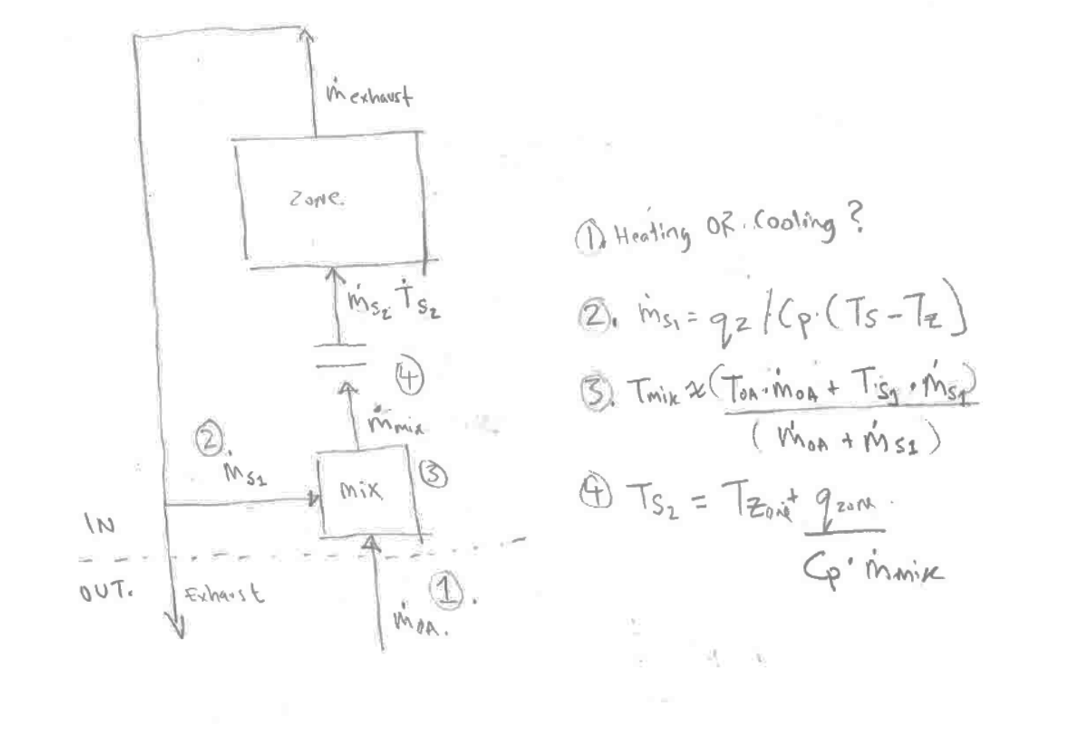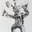Question-and-Answer Resource for the Building Energy Modeling Community
Get started with the Help page

# Clarification on Outdoor, Mixed, Supply, Exhaust Flow Rates and Temperatures in EnergyPlus Ideal Loads Air System

For my own understanding, I've recently been trying to understand how air is conditioned in an ideal load air system in EnergyPlus. Specifically, I’d like to understand how and in what order the system determines it's mass flow rates and related temperatures. I've been reading through engineering reference on the subject but am a little confused about a couple of points and wanted to get some clarification.

I've tried to write out the sequence of events here (with attached diagram), for a hypothetical scenario where heating/cooling is required. For simplicity's sake I've ignored dehumidification or humidification. I would appreciate confirmation if this overall sequenced I've written out is correct. I also have some specific questions for specific steps that I've bolded below regarding the ambiguous use of the term ‘supply air’ in the Engineering Reference.

Reference diagram for sequence outlined below:1. The ideal air loads system checks to see if the outdoor air flow rate (m_oa in diagram), specified by the ventilation rate, will require the system to be in heating, cooling or economizer mode, based on the zone setpoint conditions and sensible load brought in by the outdoor air. The temperature of this air stream is the outdoor drybulb.

2. If heating/cooling is required, the air mass flow rate for supply air (m_s1) is calculated, based on an assumed supply temperature (T_s1). The ASSUMED supply temperature is the maximum heating or minimum cooling temperature (based on equation 2).

Question 1: In the Engineering reference there are two references to a “supply air stream” with this being the first one. I’m not clear where this first supply rate is in the sequence of events, and where the mass flow rate is calculated. Is it correct to assume this mass air flow rate is applied to the air being recirculated from the exhaust airstream, before being mixed? And if so, is the actual temperature of this “supply” air the temperature of the exhaust air stream?

1. The outdoor air stream (m_oa), and the calculated supply air stream (m_s1) are mixed (m_mix = m_oa + m_s1). Heat recovery is applied if selected. I believe that the temperature/enthalpy of the mixed air stream is approximately the average of both, weighted by the mass air flows (equation 3).

2. The supply temperature for the zone is now calculated based on the resulting mixed air stream flow rate, the current zone temperature, and the sensible load required for the zone to meet it's setpoint (based on equation 4).

Question 2: Is the supply temperature here achieved via the coil in the ideal VAV terminal?

1. The supply air stream is now introduced to the zone, mixed with the room air accumulating or losing heat in accordance with specific zone loads. Then it leaves via the Zone Exhaust node and is exhausted or recirculated back to step 1.

Thanks,

S

==== REVISED BASED ON COMMENTS BELOW ====

MJWitte, I think I understand your clarification. My error is in confusing the ...

edit retag close merge delete

Thanks for the detailed explanation @MJWitte and @saeranv. How is the heat delivered to the zone when there is not Heat Recovery nor Outdoor Air? Im confused with the comparison of an Ideal Air system with/without Heat Recovery when there is not Outdoor Air. - With HR without OA, internal air is recirculated and heated up. - Without HR nor OA, how is the heat delivered and reported in the outputs?

I´m reading the Zone Ideal Loads Zone Total Heating Energy which seems to report the Heat delivered to the zone. Is the HR factored in those results?

It there is no outdoor air, all of the air is recirculated from the zone, so there cannot be any heat recovery.

Sort by » oldest newest most votedQ1: All references to supply air refer to the air supplied to the zone, S2 in your diagram. S1 in your diagram is the recirculation air. The steps in your description above are not correct. If I'm following correctly, the confusion comes from calculating the supply air mass flow rate multiple ways during the process, because it may be governed by the outdoor air flow or by one of the temperature limits. It is determined both ways and the greatest is used. Ultimately, there is only one supply air mass flow rate - that is what enters the zone. This is what the engineering reference steps are trying to describe.

Q2: Yes, sort of. There is no coil model in the ideal loads system. The change in enthalpy (broken into sensible and latent portions) between the mixed air and supply air conditions with the supply air mass flow rate are used to calculate heating/cooling loads which are reported and metered as district heating/cooling.

more